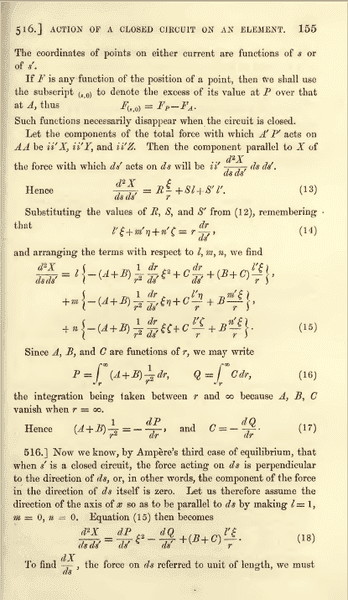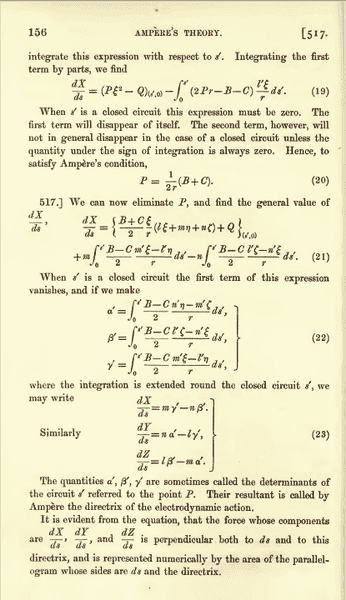# Confusion in Maxwell's derivation of Ampere's Force Law

Hi everyone here. I have my question in the following attached pdf file

#### Attachments

• confusion ampere.pdf
600.8 KB · Views: 258

Sorry I didn't posted my question directly. It was because of unavailability of Maths symbols

robphy
Homework Helper
Gold Member
This site supports ##\LaTeX## ...
enclose your expression (say) \xi\nu with opening double-# and closing double-# to produce ##\xi\nu##

I am reading Maxwell's "a treatise on electricity and magnetism, Volume 2, page 156" about "Ampere's Force Law". I have some confusion in the following pages:My question is of two parts:
1.
Equation 20, i.e. ##P=\dfrac{B+C}{2r}## is the outcome of special case (i.e. l=1, m=0, n=0)

But in Page 156, Article 517, Maxwell says: "We can now eliminate P, and find the general value of ##\dfrac{dX}{ds}## and uses this formula (i.e. ##P=\dfrac{B+C}{2r}##) in the general case.

However in the general case, where 0 < l, m, n < 1, and hence
##\dfrac{d^{2}X}{dsds'}=l\left( \frac{dP}{ds'}\xi^{2}-\dfrac{dQ}{ds'}+(B+C)\dfrac{l'\xi}{r}\right) +m(...)+n(...)\neq0##
(since direction of X is not in the direction of ds)

therefore,
##\dfrac{dX}{ds}=l\left[ (P\xi^{2}-Q)_{(s',0)}-\int\limits_0^s' (2Pr-B-C)\dfrac{l'\xi}{r}ds'\right] +m\int\limits_0^s'(...)ds'+n\int\limits_0^s'(...)ds'##
Now in this general case, how can we get ##P=\dfrac{B+C}{2r}##.

If ##P\neq\dfrac{B+C}{2r}## in general case, what does Maxwell mean by "We can now eliminate P, and find the general value of ##\dfrac{dX}{ds}##"
2. How can one get equation 21 from equation 15. Please give a lengthy derivation.